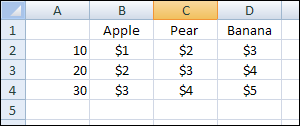## Ultimate Index: combine (nest) INDEX and MATCH..1. INDEX formula:
=INDEX(RANGE,ROWnumber,COLUMNnumber)

So in the example above, to be able see the price for 20 apples, you use:
=INDEX(B2:D4,2,1) ==> Result is \$2

2. MATCH formula:
=MATCH(LOOKUPValue,LOOKUPArray,MATCHType)

So in the example above, to know the column for Apple, you use:
=MATCH(“Apple”,B1:D1,0) ==> Result is 1

3. Using names:
Using names makes the formula easy to read, and very flexible. If your data sheet gets more columns or rows, it doesn’t matter if you set up the formula like this:

Define names: (insert, name, define)